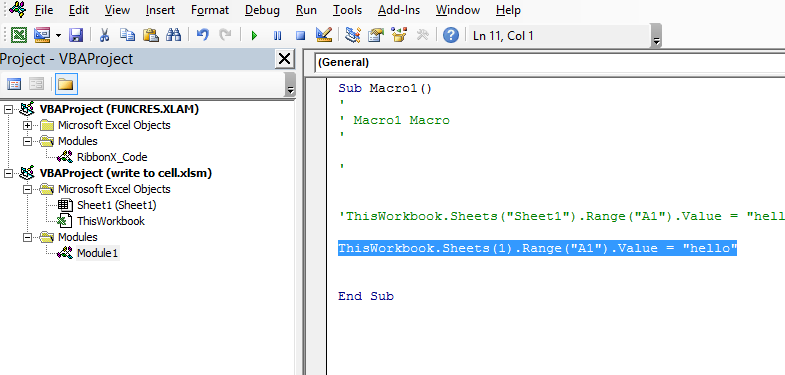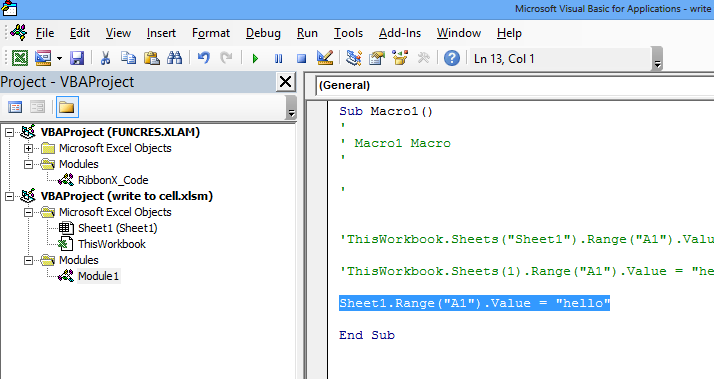### How To Write To A Cell Using Excel VBA

In this article I will guide you the basics of Excel VBA and how we can use Excel VBA to write to a cell in an Excel sheet.

Lets start from recording macro. Go to the Ribbon to the Developer tab. Click Record Marco button. Then click Edit. It will open the recorded macro as macro1 already created:Now under this module we can learn how to write any information to a cell say cell A1 of sheet1. Here are following way we can update value in cell:

### Writing to a cell using worksheet

`ThisWorkbook.Sheets("Sheet1").Range("A1").Value = "hello"`Here “Sheet1” is the name of the sheet which we have used.

Run this macro:### Writing to a cell using index number of sheet

`ThisWorkbook.Sheets(1).Range("A1").Value = "hello"`

Here we are referencing the sheet using the index which is 1 for sheet1. We can also check the total count of sheets in a workbook using sheets.count### Writing to a cell using code name

`Sheet1.Range("A1").Value = "hello"`

We are directly using the sheet1 which is the sheet name here### Writing to a cell using cells

` ThisWorkbook.Sheets(1).Cells(1, 1).Value = "hello"`

Instead of the range we are using cells (row no,column no) where row no = 1 for first row, column no = 1 for column A## Template

```Further reading: